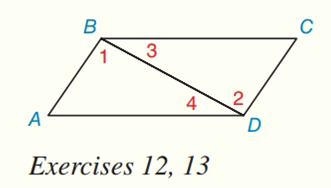Chapter 2.CT, Problem 13CT### Elementary Geometry for College St...

6th Edition
Daniel C. Alexander + 1 other
ISBN: 9781285195698

#### Solutions

Chapter
Section### Elementary Geometry for College St...

6th Edition
Daniel C. Alexander + 1 other
ISBN: 9781285195698
Textbook Problem
1 views

# If m ∠ 1 = x + 28 and m ∠ 2 = 2 x − 26 find the value x for which it follows that A B ¯ ∥ D C ¯ ._________________To determine

To find:

The value of x.

Explanation

Given:

The following figure shows the given diagram.

m1=(x+28) and m2=(2x26)

ABDC

Approach:

If two lines are parallel then the alternate interior angles will be congruent.

Calculation:

ABDC, then the alternate interior angles will be congruent that means,

m1=m2<

### Still sussing out bartleby?

Check out a sample textbook solution.

See a sample solution

#### The Solution to Your Study Problems

Bartleby provides explanations to thousands of textbook problems written by our experts, many with advanced degrees!

Get Started

#### In Problems 29-36, find the particular solution to each differential equation. when

Mathematical Applications for the Management, Life, and Social Sciences

#### limx(lnx)1/x= a) 0 b) 1 c) e d)

Study Guide for Stewart's Single Variable Calculus: Early Transcendentals, 8th

#### 45 15 63 127

Study Guide for Stewart's Multivariable Calculus, 8th

#### Explain the distinction between science and pseudoscience.

Research Methods for the Behavioral Sciences (MindTap Course List)